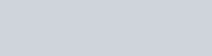A wall of length 10 m was to be built across an open ground. The height of the wall is 4m and thickness of the wall is 24 cm.
Question:

A wall of length 10 m was to be built across an open ground. The height of the wall is 4m and thickness of the wall is 24 cm. If this wall is to be built up with bricks whose dimensions are 24 cm × 12 cm × 8 cm, how many bricks would be required?

Solution:

Given that,

The wall with all its bricks makes up space occupies by it, we need to find the volume of the wall, which is nothing but cuboid.

Here, length = 10 m = 1000 cm

Thickness = 24 cm

Height = 4 m = 400 cm

Therefore, volume of the wall = l * b * h

$=1000 * 24 * 400 \mathrm{~cm}^{3}$

Now, each brick is a cuboid with length = 24 cm

Breadth = 12 cm

Height = 8 cm

So, volume of each brick $=1^{*} \mathrm{~b}^{*} \mathrm{~h}=24^{*} 12^{*} 8=2304 \mathrm{~cm}^{3}$

The number of bricks required is given by,$=\frac{1000 * 24 * 400}{2304}=4166.6$ bricks

So, the wall requires 4167 bricks.